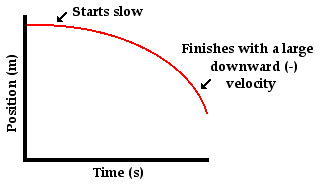1-D Kinematics - Lesson 5 - Free Fall and the Acceleration of Gravity

# Representing Free Fall by GraphsEarly in Lesson 1 it was mentioned that there are a variety of means of describing the motion of objects. One such means of describing the motion of objects is through the use of graphs - position versus time and velocity vs. time graphs. In this part of Lesson 5, the motion of a free-falling motion will be represented using these two basic types of graphs.

### Representing Free Fall by Position-Time Graphs

A position versus time graph for a free-falling object is shown below.Observe that the line on the graph curves. As learned earlier, a curved line on a position versus time graph signifies an accelerated motion. Since a free-falling object is undergoing an acceleration (g = 9.8 m/s/s), it would be expected that its position-time graph would be curved. A further look at the position-time graph reveals that the object starts with a small velocity (slow) and finishes with a large velocity (fast). Since the slope of any position vs. time graph is the velocity of the object (as learned in Lesson 3), the small initial slope indicates a small initial velocity and the large final slope indicates a large final velocity. Finally, the negative slope of the line indicates a negative (i.e., downward) velocity.

### Representing Free Fall by Velocity-Time Graphs

A velocity versus time graph for a free-falling object is shown below.Observe that the line on the graph is a straight, diagonal line. As learned earlier, a diagonal line on a velocity versus time graph signifies an accelerated motion. Since a free-falling object is undergoing an acceleration (g = 9,8 m/s/s, downward), it would be expected that its velocity-time graph would be diagonal. A further look at the velocity-time graph reveals that the object starts with a zero velocity (as read from the graph) and finishes with a large, negative velocity; that is, the object is moving in the negative direction and speeding up. An object that is moving in the negative direction and speeding up is said to have a negative acceleration (if necessary, review the vector nature of acceleration). Since the slope of any velocity versus time graph is the acceleration of the object (as learned in Lesson 4), the constant, negative slope indicates a constant, negative acceleration. This analysis of the slope on the graph is consistent with the motion of a free-falling object - an object moving with a constant acceleration of 9.8 m/s/s in the downward direction.

Next Section: